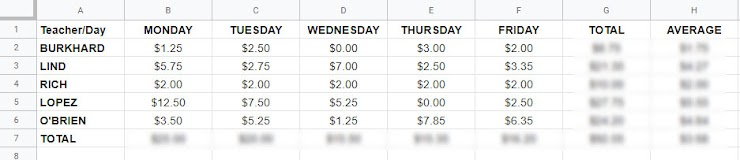MINI-PROJECT, FRESHMAN TECHNOLOGY: "The Teachers' Lunch Problem."
We have been working with Google Sheets to make charts and graphs for a while now. SHEETS can be used to complete mathematical formulas, as you know from completing the recent FORMULAS assignment.

For this mini-project, you will use Google Sheets to come up with the proper answers to the questions below, considering the following information regarding Teacher's Lunches at Highland High School.

Start a new Google Sheets file, called LUNCHES, in your FRESHMAN_TECH folder. In that file, create a table to represent the data above.

Create ROWS for each teacher and COLUMNS for each day, with extra columns on the right for Total and Average.

Then, use the SUM and AVG functions to total each teacher's lunch cost information for the day, week, on average and teacher total. See instructions from either the video tutorial or the help web page linked on the website for today! I have also included an image of a MODEL SHEET for this problem below.

When your table is complete, you can then answer the questions below.

Here is the data that you need to consider:

Mr. Burkhard spent the following amounts for lunch last week: \$1.25, 2.50, 0.00, 3.50 and 2.00.

Mr. Lind spent the following amounts for lunch last week: \$5.75, 2.75, 7.00, 2.50 and 3.35.

Mrs. Rich spent the following amounts for lunch last week: \$2.00, 2.00, 2.00, 2.00 and 2.00.

Mrs. Lopez spent the following amounts for lunch last week: \$12.50, 7.50, 5.25, 0.00 and 2.50.

Mr. O'Brien spent the following amounts for lunch last week: \$3.50, 5.25, 1.25, 7.85 and 6.35.
SAMPLE IMAGE OF TEACHER'S LUNCH SPREADSHEETSTART BY reviewing the two minute video on the webpage for today or the linked help sheet, which both outline how to insert SUM and AVERAGE formulas into SHEETS.
Name: *
Freshman Technology Class: *
Using any resources available to you (including memory of math classes past or present), define the term "AVERAGE" as it pertains to math. *
Please put this into words that a regular person can understand!
Which teacher spent the most money on lunches last week? How much did they spend, total? *
Which teacher spent the least ON AVERAGE for lunches last week? What was their average spent per day? *
(Find AVERAGE in the same way as you find SUM, as outlined in the video!)
What was the TOTAL amount of money teachers spend on lunches on Thursday? *
What was the total amount spent by all teachers last week for lunch? *
What was the AVERAGE amount PER DAY spent by all teachers for lunch last week? *
You can think of this as an AVERAGE of the AVERAGES question!